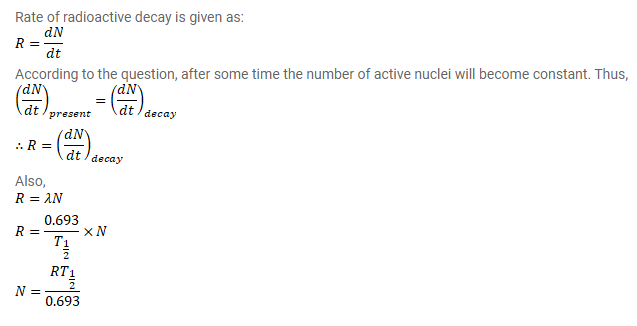# A radioactive isotope is being produced at a constant rate

Question:

A radioactive isotope is being produced at a constant rate $\mathrm{dN} / \mathrm{dt}=\mathrm{R}$ in an experiment. The isotope has a half-life $\mathrm{t}_{1 / 2}$. Show that after a time $t>t_{1 / 2}$ the number of active nuclei will become constant. Find the value of this constant.

Solution: